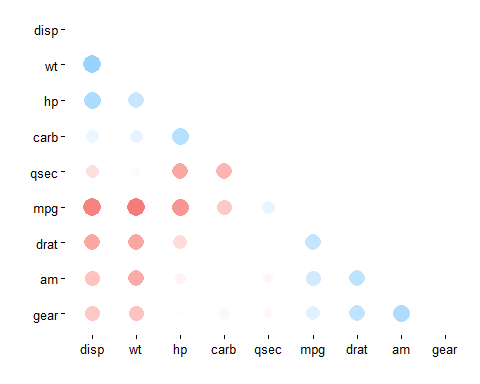# Explore correlations in R with corrr

Earlier this week, my first package, `corrr`, was made available on CRAN. Below are the introductory instructions provided on the README for this first-release version 0.1.0. Please contribute to `corrr` on Github or email me your suggestions!

## corrr #

corrr is a package for exploring correlations in R. It makes it possible to easily perform routine tasks when exploring correlation matrices such as ignoring the diagonal, focusing on the correlations of certain variables against others, or rearranging and visualising the matrix in terms of the strength of the correlations.

You can install:

• the latest released version from CRAN with
``````install.packages("corrr")
``````
• the latest development version from github with
``````if (packageVersion("devtools") < 1.6) {
install.packages("devtools")
}
devtools::install_github("drsimonj/corrr")
``````

## Using corrr #

Using `corrr` starts with `correlate()`, which acts like the base correlation function `cor()`. It differs by defaulting to pairwise deletion, and returning a correlation data frame (`cor_df`) of the following structure:

• A `tbl` with an additional class, `cor_df`
• An extra “rowname” column
• Standardised variances (the matrix diagonal) set to missing values (`NA`) so they can be ignored.

### API #

The corrr API is designed with data pipelines in mind (e.g., to use `%>%` from the magrittr package). After `correlate()`, the primary corrr functions take a `cor_df` as their first argument, and return a `cor_df` or `tbl` (or output like a plot). These functions serve one of three purposes:

Internal changes (`cor_df` out):

• `shave()` the upper or lower triangle (set to NA).
• `rearrange()` the columns and rows based on correlation strengths.

Reshape structure (`tbl` or `cor_df` out):

• `focus()` on select columns and rows.
• `stretch()` into a long format.

Output/visualisations (console/plot out):

• `fashion()` the correlations for pretty printing.
• `rplot()` plots the correlations.

## Examples #

``````library(MASS)
library(corrr)
set.seed(1)

# Simulate three columns correlating about .7 with each other
mu <- rep(0, 3)
Sigma <- matrix(.7, nrow = 3, ncol = 3) + diag(3)*.3
seven <- mvrnorm(n = 1000, mu = mu, Sigma = Sigma)

# Simulate three columns correlating about .4 with each other
mu <- rep(0, 3)
Sigma <- matrix(.4, nrow = 3, ncol = 3) + diag(3)*.6
four <- mvrnorm(n = 1000, mu = mu, Sigma = Sigma)

# Bind together
d <- cbind(seven, four)
colnames(d) <- paste0("v", 1:ncol(d))

# Insert some missing values
d[sample(1:nrow(d), 100, replace = TRUE), 1] <- NA
d[sample(1:nrow(d), 200, replace = TRUE), 5] <- NA

# Correlate
x <- correlate(d)
class(x)
#>  "cor_df"     "tbl_df"     "tbl"        "data.frame"
x
#> # A tibble: 6 x 7
#>   rowname            v1          v2           v3            v4          v5
#>     <chr>         <dbl>       <dbl>        <dbl>         <dbl>       <dbl>
#> 1      v1            NA  0.70986371  0.709330652  0.0001947192 0.021359764
#> 2      v2  0.7098637068          NA  0.697411266 -0.0132575510 0.009280530
#> 3      v3  0.7093306516  0.69741127           NA -0.0252752456 0.001088652
#> 4      v4  0.0001947192 -0.01325755 -0.025275246            NA 0.421380212
#> 5      v5  0.0213597639  0.00928053  0.001088652  0.4213802123          NA
#> 6      v6 -0.0435135083 -0.03383145 -0.020057495  0.4424697437 0.425441795
#> # ... with 1 more variables: v6 <dbl>
``````

As a `tbl`, we can use functions from data frame packages like `dplyr`, `tidyr`, `ggplot2`:

``````library(dplyr)

# Filter rows by correlation size
x %>% filter(v1 > .6)
#> # A tibble: 2 x 7
#>   rowname        v1        v2        v3          v4          v5
#>     <chr>     <dbl>     <dbl>     <dbl>       <dbl>       <dbl>
#> 1      v2 0.7098637        NA 0.6974113 -0.01325755 0.009280530
#> 2      v3 0.7093307 0.6974113        NA -0.02527525 0.001088652
#> # ... with 1 more variables: v6 <dbl>
``````

corrr functions work in pipelines (`cor_df` in; `cor_df` or `tbl` out):

``````x <- datasets::mtcars %>%
correlate() %>%    # Create correlation data frame (cor_df)
focus(-cyl, -vs, mirror = TRUE) %>%  # Focus on cor_df without 'cyl' and 'vs'
rearrange(method = "HC", absolute = FALSE) %>%  # arrange by correlations
shave() # Shave off the upper triangle for a clean result

fashion(x)
#>      disp wt   hp   carb qsec mpg  drat am   gear
#> disp
#> wt    .89
#> hp    .79  .66
#> carb  .39  .43  .75
#> qsec -.43 -.17 -.71 -.66
#> mpg  -.85 -.87 -.78 -.55  .42
#> drat -.71 -.71 -.45 -.09  .09  .68
#> am   -.59 -.69 -.24  .06 -.23  .60  .71
#> gear -.56 -.58 -.13  .27 -.21  .48  .70  .79
rplot(x)
``````